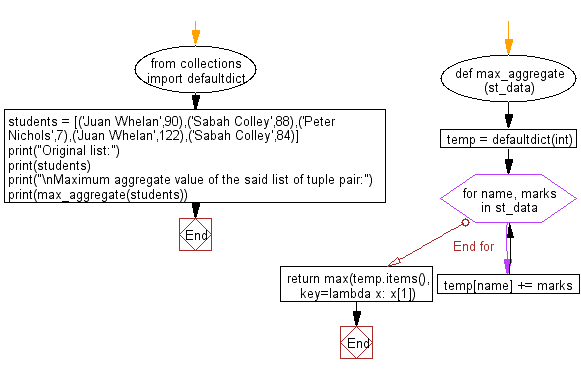﻿ Python: Calculate the maximum aggregate from the list of tuples (pairs) - w3resource# Python: Calculate the maximum aggregate from the list of tuples (pairs)

## Python Collections: Exercise-24 with Solution

Write a Python program to calculate the maximum aggregate from the list of tuples (pairs).

Sample Solution:

Python Code:

``````from collections import defaultdict
def max_aggregate(st_data):
temp = defaultdict(int)
for name, marks in st_data:
temp[name] += marks
return max(temp.items(), key=lambda x: x)

students = [('Juan Whelan',90),('Sabah Colley',88),('Peter Nichols',7),('Juan Whelan',122),('Sabah Colley',84)]
print("Original list:")
print(students)
print("\nMaximum aggregate value of the said list of tuple pair:")
print(max_aggregate(students))
```
```

Sample Output:

```Original list:
[('Juan Whelan', 90), ('Sabah Colley', 88), ('Peter Nichols', 7), ('Juan Whelan', 122), ('Sabah Colley', 84)]

Maximum aggregate value of the said list of tuple pair:
('Juan Whelan', 212)
```

Flowchart:## Visualize Python code execution:

The following tool visualize what the computer is doing step-by-step as it executes the said program:

Python Code Editor:

Have another way to solve this solution? Contribute your code (and comments) through Disqus.

What is the difficulty level of this exercise?

Test your Programming skills with w3resource's quiz.

﻿

## Python: Tips of the Day

Use Reversed() In for Loops:

```>>> tasks = ['laundry', 'picking up kids', 'gardening', 'cooking']